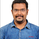4068 뷰
Uses two sets of MACD plus an RSI to either long or short. All three indicators trigger buy/sell as one ( ie it's not 'IF MACD1 OR MACD2 OR RSI > 1 = buy", its more like "IF 1 AND 2 AND RSI=buy", all 3 match required for trigger)

The MACD inputs should be tweaked depending on timeframe and what you are trading. If you are doing 1, 3, 5 min or real frequent trading then 21/44/20 and 32/66/29 or other high value MACDs should be considered. If you are doing longer intervals like 2, 3, 4hr then consider 9/19/9 and 21/44/20 for MACDs (experiment! I picked these example #s randomly).
Ideal usage for the MACD sets is to have MACD2 inputs at around 1.5x, 2x, or 3x MACD1's inputs.

Other settings to consider: try having fastlength1=macdlength1 and then (fastlength2 = macdlength2 - 2). Like 10/26/10 and 23/48/20. This seems to increase net profit since it is more likely to trigger before major price moves, but may decrease profitable trade %. Conversely, consider FL1=MCDL1 and FL2 = MCDL2 + (FL2 * 0.5). Example: 10/26/10 and 22/48/30 this can increase profitable trade %, though may cost some net profit.

Feel free to message me with suggestions or questions.
오픈 소스 스크립트

진정한 TradingView의 정신에 따라, 이 스크립트의 작성자는 스크립트를 오픈소스로 게시했기에 거래자들이 이해하고 확인할 수 있습니다. 작가님께 건배! 스크립트를 무료로 사용할 수 있지만, 게시물에서 이 코드를 재사용하는 것은 하우스룰을 따릅니다. 당신은 스크립트를 차트에 사용하기 위해 그것을 즐겨찾기 할 수 있습니다.

차트에 이 스크립트를 사용하시겠습니까?
```//@version=2
strategy("MACDbl RSI", overlay=true)

fastLength = input(10)
slowlength = input(22)
MACDLength = input(9)

MACD = ema(close, fastLength) - ema(close, slowlength)
aMACD = sma(MACD, MACDLength)
delta = MACD - aMACD

fastLength2 = input(21)
slowlength2 = input(45)
MACDLength2 = input(20)

MACD2 = ema(open, fastLength2) - ema(open, slowlength2)
aMACD2 = sma(MACD2, MACDLength2)
delta2 = MACD2 - aMACD2

Length = input(14, minval=1)
Oversold = input(20, minval=1)
Overbought = input(70, minval=1)
xRSI = rsi(open, Length)

if (delta > 0) and (year>2015) and (delta2 > 0) and (xRSI < Overbought)

if (delta < 0) and (year>2015) and (delta2 < 0) and (xRSI > Oversold)
strategy.entry("sell", strategy.short, comment="sell")

//plot(strategy.equity, title="equity", color=red, linewidth=2, style=areabr)```

## 코멘트Hi Ryan

I am newbie, how i can execute this script and get this trigger?
응답Kalghamdi12
from B<E ? is that an abbreviation of what the error message showed up or what it said verbatim? I couldn't get it to reproduce on my end. Perhaps it was the symbol you were looking up it somehow didn't like. If you give me the specifics of what symbol and time interval you were using I'll see if I can help.
응답